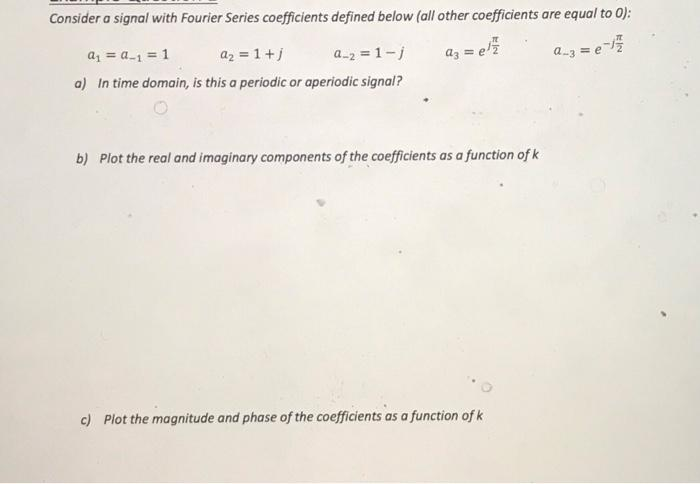Home / Expert Answers / Electrical Engineering / consider-a-signal-with-fourier-series-coefficients-defined-below-all-other-coefficients-are-equal-t-pa660

# (Solved): Consider a signal with Fourier Series coefficients defined below (all other coefficients are equal t ...

Consider a signal with Fourier Series coefficients defined below (all other coefficients are equal to 0): a3 = e/²7/2 -17 a₁ = a_₁ = 1 a₂ = 1 +j a_2 = 1-j a) In time domain, is this a periodic or aperiodic signal? b) Plot the real and imaginary components of the coefficients as a function of k c) Plot the magnitude and phase of the coefficients as a function of k a_3 = eConsider a signal with Fourier Series coefficients defined below (all other coefficients are equal to 0): a) In time domain, is this a periodic or aperiodic signal? b) Plot the real and imaginary components of the coefficients as a function of c) Plot the magnitude and phase of the coefficients as a function of

We have an Answer from Expert